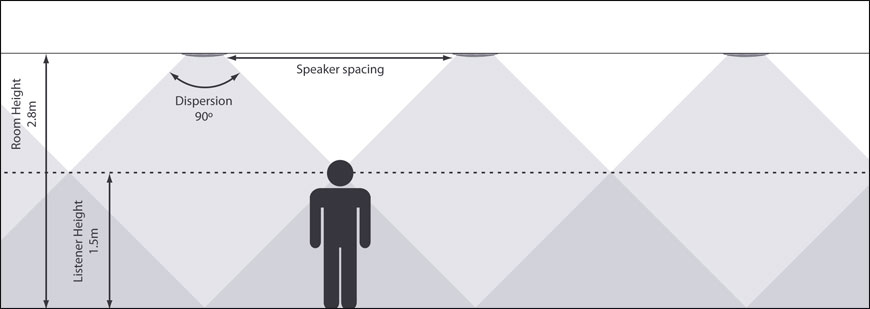# Dispersion angles

Calculating the spacing required in-between speakers using the dispersion angle. The spacing between speakers should be the distance. Calculating the spacing requires trigonometry between the dispersion overlap at the listener’s height. (using Tangent) in the following equation.

(speaker height – listener height) × TAN (dispersion angle ÷ 2) × 2 = separation distance

Example :
For a speaker with 90° dispersion angle in a 2.8m high room and assuming that an average person is around 1.5m tall

= (2.8 – 1.5) × TAN (45°) × 2
= 1.3 x 1 x 2 = 2.6

Therefore, speakers should be spaced 2.6m apart